Choose Language Hide Translation Bar
Highlighted

## How can I import the column property into JMP data file?

Hi,

New challenge:

I've created the Column Property: Test Number in the 1st_file. Each test has unique Test Number which can be found in the column property as you can see below in highlighted red circle: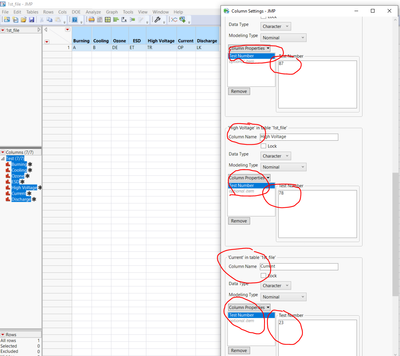I've a 2nd JMP file which has 1 column(Test):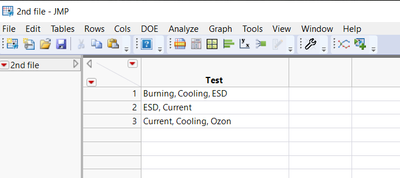I'm  trying to write a script which can copy the Test Number from the 1st file and assign the lowest value among of the Test in the 2nd file so my output will relatively look like this:

The Test Number are defined in the Column property of 1st file

E.g. Burning, Cooling, ESD = Min[Test Number] = 21

Output: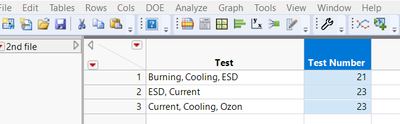Any suggestions?

Thanks

3 ACCEPTED SOLUTIONS

Accepted Solutions
Highlighted

## Re: How can I import the column property into JMP data file?

@Jacksmith12 You have been shown all of these statements and functions in previous examples.  You need to start to be able to try to put them together yourself.  In computing, the only way you learn, is by making mistakes, and figuring out what the problem is.  So below is a flow for a single entry from your 2nd_file, that returns the minimum test number.  You should be able to put remainder of the code together.

``````names default to here(1);

dt1 = data table("1st_file ");
dt2 = data table("2nd file ");

dt2 << New Column("Test Number");

theWord = word(1,dt2:test,",");

theTest = column( dt1, theWord ) << get property("Test Number");

theWord = word(2,dt2:test,",");

theCompare = column( dt1, theWord ) << get property("Test Number");

If( theCompare < theWord, theTest = theCompare );

theWord = word(3,dt2:test,",");

theCompare = column( dt1, theWord ) << get property("Test Number");

If( theCompare < theWord, theTest = theCompare );

dt2:test number = theTest;``````

Make sure you understand each line of the code, and if you do not, then ask questions specifically about what you do not understand.

Jim
Highlighted

## Re: How can I import the column property into JMP data file?

``````Names Default To Here( 1 );

dt1 = Data Table( "1st_file" );
dt2 = Data Table( "2nd file" );

For( i = 1, i <= N Rows( dt2 ), i++,

k = 1;
While( Word( k, dt2:test[i], "," ) != "",

theWord = Word( k, dt2:test[i], "," );

If( k == 1,
theTest = Column( dt1, theWord ) << get property( "Test Number" ),
theCompare = Column( dt1, theWord ) << get property( "Test Number" );

If( theCompare < theTest,
theTest = theCompare
);
);
k++;
);

dt2:test number[i] = theTest;
);``````
Jim
Highlighted

## Re: How can I import the column property into JMP data file?

Good try on the If.....but the branching needs to be larger in scope.  See below......

``````Names Default To Here( 1 );

dt1 = Data Table( "1st_file" );
dt2 = Data Table( "2nd file" );

dt2 << New Column( "Test Number" );

For( i = 1, i <= N Rows( dt2 ), i++,
k = 1;
While( Word( k, dt2:test[i], "," ) != "",

theWord = Word( k, dt2:test[i], "," );
If( theWord != "N/A",
If( k == 1,
theTest = Column( dt1, theWord ) << get property( "Test Number" ),
theCompare = Column( dt1, theWord ) << get property( "Test Number" );

If( theCompare < theTest,
theTest = theCompare
);
)
,
theTest = 0
);
k++;
);

dt2:test number[i] = theTest;
);``````
Jim
15 REPLIES 15
Highlighted

## Re: How can I import the column property into JMP data file?

How does one know, which of the columns in 1st_file are evaluated in the calculation of a particular row's value in 2nd_file?
Jim
Highlighted

## Re: How can I import the column property into JMP data file?

Hi @txnelson,

Right click on column properties and you will find Test Number and the Test Name

Highlighted

## Re: How can I import the column property into JMP data file?

In 1st_file, the columns only have 1 Column Property each, Test Number.....no Test Name.
Assuming that a second Column Property for each column of Test Name should have been in the 1st_File data table, then I believe the best way to access them would be to create an Associative Array. Then loop through each of the columns, extracting the 2 column properties, and placing them into the Associative Array. Finally, one would loop through the 2nd_file, row by row, and taking the value of the column Test, it would be a simple lookup against the Associative Array, to get the required test number.
Jim
Highlighted

## Re: How can I import the column property into JMP data file?

Can you give a quick start example?
Highlighted

## Re: How can I import the column property into JMP data file?

@Jacksmith12 You have been shown all of these statements and functions in previous examples.  You need to start to be able to try to put them together yourself.  In computing, the only way you learn, is by making mistakes, and figuring out what the problem is.  So below is a flow for a single entry from your 2nd_file, that returns the minimum test number.  You should be able to put remainder of the code together.

``````names default to here(1);

dt1 = data table("1st_file ");
dt2 = data table("2nd file ");

dt2 << New Column("Test Number");

theWord = word(1,dt2:test,",");

theTest = column( dt1, theWord ) << get property("Test Number");

theWord = word(2,dt2:test,",");

theCompare = column( dt1, theWord ) << get property("Test Number");

If( theCompare < theWord, theTest = theCompare );

theWord = word(3,dt2:test,",");

theCompare = column( dt1, theWord ) << get property("Test Number");

If( theCompare < theWord, theTest = theCompare );

dt2:test number = theTest;``````

Make sure you understand each line of the code, and if you do not, then ask questions specifically about what you do not understand.

Jim
Highlighted

## Re: How can I import the column property into JMP data file?

@txnelson You are genius. Yes you are correct I will start learning. Thanks a lot
Highlighted

## Re: How can I import the column property into JMP data file?

Hi @txnelson ,

I used For loop in the script which you posted.

``````names default to here(1);

dt1 = data table("1st_file");
dt2 = data table("2nd file");

dt2 << New Column("Test Number");

For(i=1, i<=nrows(dt2),i++,

theWord = word(i,dt2:test[i],",");

theTest = column( dt1, theWord ) << get property("Test Number");

theWord = word(i,dt2:test[i],",");

theCompare = column( dt1, theWord ) << get property("Test Number");

If( theCompare < theWord, theTest = theCompare );

theWord = word(i,dt2:test[i],",");

theCompare = column( dt1, theWord ) << get property("Test Number");

If( theCompare < theWord, theTest = theCompare );

dt2:test number[i] = theTest;

);
``````

It's not assigning values for rows 4 and 5 of 2nd file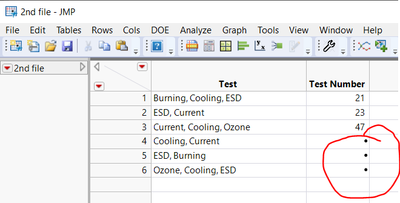I am pretty sure I made some mistake in For loop. Can you please provide me some guidance.

Highlighted

## Re: How can I import the column property into JMP data file?

The issue is with this statement

``theWord = word(i,dt2:test[i],",");``

Your For() loop is using "i" as it's index, and it varies from 1 to N Rows(dt2).  Therefore it varies from 1 to 5.  You are using the "i" index in 2 different places within the Word() function.  dt2:Test[i] will read the 1st through 5 rows.  But, if you take a look at the JSL Syntax Reference for the Word() function

```Word(n|[first last], string, <delimiter>, <Unmatched(result string)>)
Description
Returns the nth item of the string, where words are sub-strings separated by any number of
any characters in the delimiter argument.
Arguments
n The position of the word being extracted.
[first last] A matrix that defines the beginning and end word range to return.
string The string that is evaluated.
delimiter (Optional) The character used as a boundary. If delimiter is absent, an ASCII
space is used. If delimiter is the empty string, each character is treated as a separate
word. If delimiter is an empty string, each character is treated as a spate word.
Unmatched(result string) The string to print if no match is found.
Examples
This example returns the last name:
Word( 2, "Katie Layman" );
Note
See “Item(n|[first last], string, <delimiter>, <Unmatched(result string)>, <Include
Boundary Delimiters(Boolean)>)” on page 35 for examples of how Word() differs from
Item().```

what you are specifying for the Word you want to strip off is dependent on the value of "i".  That is not what you want.  In the code I provided, if you look, it was either a 1,2, or 3

So you need to adjust that

Jim
Highlighted

## Re: How can I import the column property into JMP data file?

Hi @txnelson,

The logic which you suggested doesn't work for some conditions. If I change the order of the Test the script generates incorrect values and the Test number for rows 4,5,6 are blank

Test = Cooling, ESD, Current

Test Number = Minimum of Test Number[32, 87, 23] = 23

The Test Number should be 23 in the 2nd Column.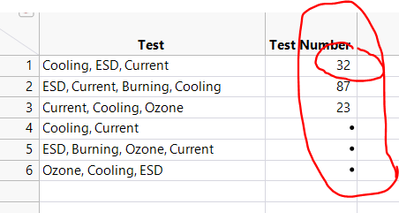I corrected the statement which you suggested :

``````For (i=1, i<=nrows(dt2), i++,
theWord = word(1,dt2:test[i],",");

theTest = column( dt1, theWord ) << get property("Test Number");

theWord = word(2,dt2:test[i],",");

theCompare = column( dt1, theWord ) << get property("Test Number");

If( theCompare < theWord, theTest = theCompare );

theWord = word(3,dt2:test[i],",");

theCompare = column( dt1, theWord ) << get property("Test Number");

If( theCompare < theWord, theTest = theCompare );

dt2:test number[i] = theTest;
);``````

Article Labels

There are no labels assigned to this post.